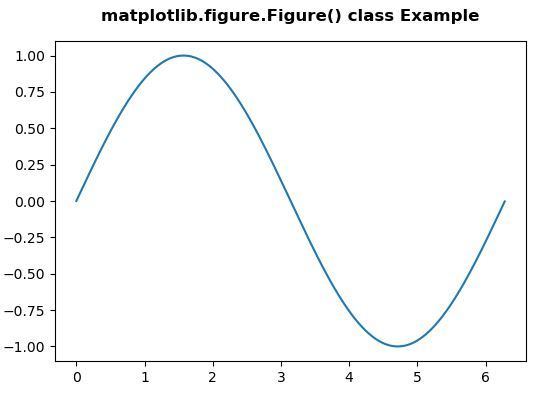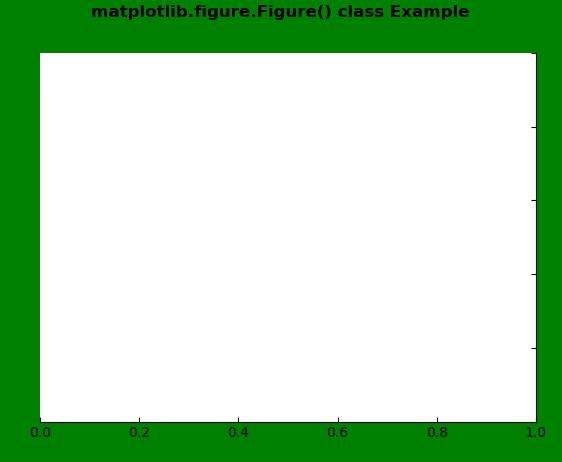Related Articles
Matplotlib.figure.Figure() in Python
• Last Updated : 30 Apr, 2020

Matplotlib is a library in Python and it is numerical – mathematical extension for NumPy library. The figure module provides the top-level Artist, the Figure, which contains all the plot elements. This module is used to control the default spacing of the subplots and top level container for all plot elements.

## matplotlib.figure.Figure() class

This class is the top level container for all the plot elements.The Figure instance supports callbacks through a callbacks attribute which is a CallbackRegistry instance.
Note: In the Figure, the Base is the matplotlib.artist.Artist.

Syntax: class matplotlib.figure.Figure(figsize=None, dpi=None, facecolor=None, edgecolor=None, linewidth=0.0, frameon=None, subplotpars=None, tight_layout=None, constrained_layout=None)

Parameters: This accept the following parameters that are described below:

• figsize : This parameter is the Figure dimension (width, height) in inches.
• dpi : This parameter is the dots per inch.
• facecolor : This parameter is the figure patch facecolor.
• edgecolor : This parameter is the figure patch edge color.
• linewidth : This parameter is the linewidth of the frame.
• frameon : This parameter is the suppress drawing the figure background patch.
• subplotpars : This parameter is the Subplot parameters.
• tight_layout : This parameter is used to adjust subplot parameters.
• constrained_layout : This parameter is used to adjust positioning of plot elements.

Returns: This method return the figure instances.

Below examples illustrate the matplotlib.figure.Figure() function in matplotlib.figure:

Example 1:

 `# Implementation of matplotlib function ``import` `matplotlib.pyplot as plt ``from` `matplotlib.figure ``import` `Figure``import` `numpy as np ``   ` ` ` `fig ``=` `plt.figure(figsize ``=``(``5``, ``4``)) ``   ` `ax ``=` `fig.add_axes([``0.1``, ``0.1``, ``0.8``, ``0.8``])`` ` `xx ``=` `np.arange(``0``, ``2` `*` `np.pi, ``0.01``) ``ax.plot(xx, np.sin(xx)) ``   ` `fig.suptitle(``'matplotlib.figure.Figure() class Example\n\n'``,  ``             ``fontweight ``=``"bold"``) ``   ` `plt.show() `

Output:Example-2:

 `# Implementation of matplotlib function ``import` `matplotlib.pyplot as plt ``from` `matplotlib.figure ``import` `Figure``from` `mpl_toolkits.axisartist.axislines ``import` `Subplot ``import` `numpy as np ``  ` ` ` `fig ``=` `plt.figure(facecolor ``=``"green"``) ``   ` `ax ``=` `Subplot(fig, ``111``) ``fig.add_subplot(ax) ``   ` `ax.axis[``"left"``].set_visible(``False``) ``ax.axis[``"top"``].set_visible(``False``)`` ` `fig.suptitle(``'matplotlib.figure.Figure() class Example\n\n'``,  ``             ``fontweight ``=``"bold"``) ``   ` `plt.show() `

Output:Attention geek! Strengthen your foundations with the Python Programming Foundation Course and learn the basics.

To begin with, your interview preparations Enhance your Data Structures concepts with the Python DS Course. And to begin with your Machine Learning Journey, join the Machine Learning – Basic Level Course

My Personal Notes arrow_drop_up